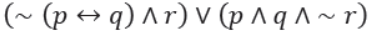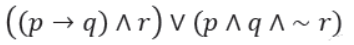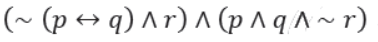﻿ GATE CS Applied Course

## GATE Previous Subject Wise Questions

##### Question 141
An operating system uses the Banker’s algorithm for deadlock avoidance when managing the allocation of three resource types X, Y, and Z to three processes P0, P1, and P2. The table given below presents the current system state. Here, the Allocation matrix shows the current number of resources of each type allocated to each process and the Max matrix shows the maximum number of resources of each type required by each process during its execution.There are 3 units of type X, 2 units of type Y and 2 units of type Z still available. The system is currently in a safe state. Consider the following independent requests for additional resources in the current state:
REQ1: P0 requests 0 units of X,
0 units of Y and 2 units of Z
REQ2: P1 requests 2 units of X,
0 units of Y and 0 units of Z
Which one of the following is TRUE?

A
Only REQ1 can be permitted.
B
Only REQ2 can be permitted.
C
Both REQ1 and REQ2 can be permitted
D
Neither REQ1 nor REQ2 can be permitted
operating systems GATE 2014 SET 1
##### Question 142
Consider the following set of processes that need to be scheduled on a single CPU. All the times are given in milliseconds.
Process Name      Arrival Time      Execution Time
A                  0                  6
B                  3                  2
c                  5                  4
D                  7                  6
E                  10                 3
Using the shortest remaining time first scheduling algorithm, the average process turnaround time (in msec) is ____________________.
Submit
operating systems GATE 2014 SET 1
##### Question 143
Assume that there are 3 page frames which are initially empty. If the page reference string is 1, 2, 3, 4, 2, 1, 5, 3, 2, 4, 6, the number of page faults using the optimal replacement policy is__________.
Submit
operating systems GATE 2014 SET 1
##### Question 144
The minimum number of comparisons required to find the minimum and the maximum of 100 numbers is ______________.
Submit
Algorithms GATE 2014 SET 1
##### Question 145
Consider a hash table with 9 slots. The hash function is ?(k) = k mod 9. The collisions are resolved by chaining. The following 9 keys are inserted in the order: 5, 28, 19, 15, 20, 33, 12, 17, 10. The maximum, minimum, and average chain lengths in the hash table, respectively, are
A
3, 0, and 1
B
3, 3, and 3
C
4, 0, and 1
D
3, 0, and 2
Data Structures GATE 2014 SET 1 Hashing
##### Question 146
A pennant is a sequence of numbers, each number being 1 or 2. An n-pennant is a sequence of numbers with sum equal to n. For example, (1,1,2) is a 4-pennant. The set of all possible 1-pennants is {(1)}, the set of all possible 2-pennants is {(2), (1,1)} and the set of all 3-pennants is {(2,1), (1,1,1), (1,2)}. Note that the pennant (1,2) is not the same as the pennant (2,1). The number of 10- pennants is ______________.
Submit
GATE 2014 SET 1
##### Question 147
Let S denote the set of all functions f: {0,1}4 -> {0,1}. Denote by N the number of functions from S to the set {0,1}. The value of Log2Log2N is ______.
Submit
GATE 2014 SET 1
##### Question 148
An ordered n-tuple (d1, d2, … , dn) with d1 >= d2 >= ? >= dn is called graphic if there exists a simple undirected graph with n vertices having degrees d1, d2, … , dn respectively. Which of the following 6-tuples is NOT graphic?
A
(1, 1, 1, 1, 1, 1)
B
(2, 2, 2, 2, 2, 2)
C
(3, 3, 3, 1, 0, 0)
D
(3, 2, 1, 1, 1, 0)
GATE 2014 SET 1
##### Question 149
Which one of the following propositional logic formulas is TRUE when exactly two of p, q, and r are TRUE?
ABCDGATE 2014 SET 1
##### Question 150
Given the following schema:
     employees(emp-id, first-name, last-name, hire-date, dept-id, salary)
departments(dept-id, dept-name, manager-id, location-id) 
You want to display the last names and hire dates of all latest hires in their respective departments in the location ID 1700. You issue the following query:
SQL> SELECT last-name, hire-date
FROM employees
WHERE (dept-id, hire-date) IN ( SELECT dept-id, MAX(hire-date)
FROM employees JOIN departments USING(dept-id)
WHERE location-id = 1700
GROUP BY dept-id); 
What is the outcome?
A
It executes but does not give the correct result.
B
It executes and gives the correct result.
C
It generates an error because of pairwise comparison.
D
It generates an error because the GROUP BY clause cannot be used with table joins in a subquery
GATE 2014 SET 1
##### Question 151
Consider two processors P1 and P2 executing the same instruction set. Assume that under identical conditions, for the same input, a program running on P2 takes 25% less time but incurs 20% more CPI (clock cycles per instruction) as compared to the program running on P1. If the clock frequency of P1 is 1GHz, then the clock frequency of P2 (in GHz) is _________.
Submit
computer organization GATE 2014 SET 1
##### Question 152
The maximum number of edges in a bipartite graph on 12 vertices is __________________________.
Submit
GATE 2014 SET 2
##### Question 153
A priority queue is implemented as a Max-Heap. Initially, it has 5 elements. The level-order traversal of the heap is: 10, 8, 5, 3, 2. Two new elements 1 and 7 are inserted into the heap in that order. The level-order traversal of the heap after the insertion of the elements is:
A
10, 8, 7, 3, 2, 1, 5
B
10, 8, 7, 2, 3, 1, 5
C
10, 8, 7, 1, 2, 3, 5
D
10, 8, 7, 5, 3, 2, 1
Data Structures GATE 2014 SET 2 Heap-Tree
##### Question 154
Which one of the following correctly determines the solution of the recurrence relation with T(1) = 1?
T(n) = 2T(n/2) + Logn
A
Θ(n)
B
Θ(nLogn)
C
Θ(n*n)
D
Θ(log n)
Algorithms GATE 2014 SET 2 Time-Complexity
##### Question 155
Consider the tree arcs of a BFS traversal from a source node W in an unweighted, connected, undirected graph. The tree T formed by the tree arcs is a data structure for computing.
A
the shortest path between every pair of vertices.
B
the shortest path from W to every vertex in the graph
C
the shortest paths from W to only those nodes that are leaves of T.
D
the longest path in the graph
Data Structures GATE 2014 SET 2 Graphs
##### Question 156
A FAT (file allocation table) based file system is being used and the total overhead of each entry in the FAT is 4 bytes in size. Given a 100 x 106 bytes disk on which the file system is stored and data block size is 103 bytes, the maximum size of a file that can be stored on this disk in units of 106 bytes is ____________.
Submit
operating systems GATE 2014 SET 2
##### Question 157
Consider the procedure below for the Producer-Consumer problem which uses semaphores:Which one of the following is TRUE?
A
The producer will be able to add an item to the buffer, but the consumer can never consume it.
B
The consumer will remove no more than one item from the buffer.
C
Deadlock occurs if the consumer succeeds in acquiring semaphore s when the buffer is empty.
D
The starting value for the semaphore n must be 1 and not 0 for deadlock-free operation.
operating systems GATE 2014 SET 2
##### Question 158
Three processes A, B and C each execute a loop of 100 iterations. In each iteration of the loop, a process performs a single computation that requires tc CPU milliseconds and then initiates a single I/O operation that lasts for tio milliseconds. It is assumed that the computer where the processes execute has sufficient number of I/O devices and the OS of the computer assigns different I/O devices to each process. Also, the scheduling overhead of the OS is negligible. The processes have the following characteristics:
Process id      tc      tio
A        100 ms    500 ms
B        350 ms    500 ms
C        200 ms    500 ms
The processes A, B, and C are started at times 0, 5 and 10 milliseconds respectively, in a pure time sharing system (round robin scheduling) that uses a time slice of 50 milliseconds. The time in milliseconds at which process C would complete its first I/O operation is ___________.

A
500
B
1000
C
2000
D
10000
operating systems GATE 2014 SET 2
##### Question 159
A computer has twenty physical page frames which contain pages numbered 101 through 120. Now a program accesses the pages numbered 1, 2, …, 100 in that order, and repeats the access sequence THRICE. Which one of the following page replacement policies experiences the same number of page faults as the optimal page replacement policy for this program?
A
Least-recently-used
B
First-in-first-out
C
Last-in-first-out
D
Most-recently-used
operating systems GATE 2014 SET 2
##### Question 160
Consider two strings A = "qpqrr" and B = "pqprqrp". Let x be the length of the longest common subsequence (not necessarily contiguous) between A and B and let y be the number of such longest common subsequences between A and B. Then x + 10y = ___.
A
33
B
23
C
43
D
34
Algorithms GATE 2014 SET 2 Dynamic-Programming© 2020 - All rights are reserved- AAIC Technologies pvt ltd